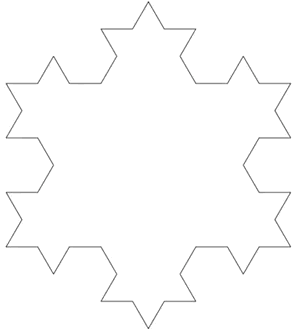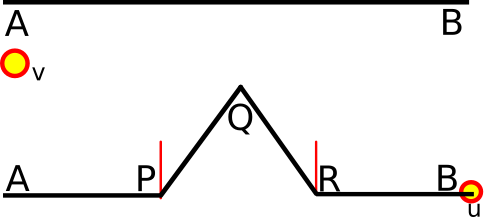Lately it’s been feeling a bit more wintery in Chicago. In honor of the fresh powder, transient though it be, let’s code a snowflake fractal that we can enjoy all year long.

## Let’s Code a Snowflake! [codepen demo]##### a Koch Snowflake going through 8 iterations from 3 to 12,288 sides

A Koch Snowflake is a fractal that is generated by the recursive subdivision of line segments according to a simple rule. For the Snowflake seen above, the initial state is three line segments that form an equilateral triangle.

The Koch Snowflake has the fascinating property that while it bounds a finite area, its perimeter tends toward infinity. Let’s code this infinite perimeter in finite time.

### Let’s define some simple objects.

We need to work with Segments. A line segment has two Points.

``````var Point = function(x, y) {
this.x = x;
this.y = y;
};
``````

Points can be added: sum the X and Y component of both points

``````Point.prototype.add = function(B) {
let newX = this.x + B.x;
let newY = this.y + B.y;
return new Point(newX, newY);
};
``````

Points can be multiplied by a scalar: multiply the X and Y component by the scalar value

``````Point.prototype.scalarMultiply = function(B) {
let newX = this.x * B;
let newY = this.y * B;
return new Point(newX, newY);
};
``````

A segment holds two points.

``````var Segment = function(args = {}) {
const { A, B } = args;
this.A = new Point(A, A);
this.B = new Point(B, B);
};

Segment.prototype.split = function() {
/* magical split function, to be defined */
}
``````

`Segment.split()` will turn a segment into four line segments per the Koch method.

### We need to do some math.##### thanks to excellent StackOverflow answer

`Segment.split()` must calculate the value of points P, Q, and R given points A and B.

We perform the following operation to create four new line segments from segment AB:

1. Convert AB into three equal parts of `length` (red lines are the two split points)
2. Draw an equilateral triangle with base points `length/3` (P) and `2*length/3` (R)
3. Remove the base of the triangle, segment PR.

To handle Step 3, we will simply not calculate the base of the triangle at all. Now we just need to calculate the X and Y coordinates of points A, B, P, Q, and R.

### A and B

A and B are trivial.

`A: [this.A.x, this.A.y]` `B: [this.B.x, this.B.y]`

### P and R

P and R are also quite simple. We create the helper point `U` which has coordinates

`[this.B.x - this.A.x, this.B.y - this.A.y]`

U helps us translate the Y component of P and R when AB is not horizontal.

We can then calculate points P and R with the `scalarMultiply` method that we gave our points. We can calculate these points relative to A. Points P and R correspond to the two red lines in the diagram, or the 1/3 and 2/3 split of the segment AB.

P: `let P = this.A.add(U.scalarMultiply(1 / 3));`

R: `let R = this.A.add(U.scalarMultiply(2 / 3));`

If the line AB is horizontal, `A.y==B.y`, so the Y component of `U` will be zero. However, if the line is at an angle relative to the X-axis, the scalar multiplication adjusts the Y-component of P and R by a factor of (1/3) and (2/3) times the “difference in Y” between A and B.

Q is the trickiest one. Just repeat: move to the midpoint of AB and distribute the height, proportional to AB’s orientation. That’s all there is to it.

Okay, sorry…that was a mouthful.

We know the altitude of an equilateral triangle is `side*sqrt(3)/2`.

Since our equilateral triangle consists of a third of the side length, the height of our triangle becomes `(side/3)*sqrt(3)/2` or `side*sqrt(3)/6`.

Q will always be altitude `side*sqrt(3)/6` away from segment AB. The question is only: how do we calculate this point when AB is not horizontal?

How much of the altitude is distributed to the X and Y component of Q, given a rotation of AB?

Here we make use of the helper point `V`, which has coordinates

`[this.A.y - this.B.y, this.B.x - this.A.x]`

Point V is the result of rotating Point U 90 degrees counterclockwise relative to the origin. You can rotate a point (x, y) in this way by transforming it into (-y, x).

Once we have Point V, we have only to use it.

V helps us translate the X component of Q when AB is not horizontal.

``````let Q = this.A
``````

First, we translate Point A to the midpoint of AB by multiplying by `U*(1/2)`.

Then we need to distribute the height to the X and Y components based on the orientation of AB.

Have a look at this diagram and work through the following three cases.1. Consider: if AB is horizontal, `A.y-B.y==0`, so the x-component of V will be zero. We distribute all the height to the Y component of Q.

2. Consider: if AB is rotated 45 degrees CCW relative to the origin, `A.y-B.y==(-1)*s/sqrt(2)` and `B.x-A.x==s/sqrt(2)`. We distribute half the height to the X component of Q (subtracting from Q) and half the height to the Y component of Q.

3. Consider: if AB is rotated 90 degrees CCW relative to the origin, `A.y-B.y==(-1*)sideLength`. `B.x-A.x==0`. We distribute all the height to the X component of Q (subtracting from Q) and none of the height to the Y component of Q (Q’s Y stays at the midpoint of AB).

Note how since we handled these cases for the first and second quadrant, where `A.y<B.y`, we are also covered if the rotation is CW about the origin into the third or fourth quadrant: in these cases, `A.y>B.y`, so we ADD to the X component of Q instead of subtract.

### We finished Segment.split()!

We already did all the tricky maths. Now we just have to fill in the points, based our calculations above. Coding Segment.split is trivial, since we planned what we were going to do before we tried to do it.

``````Segment.prototype.split = function() {
let U = new Point(this.B.x - this.A.x, this.B.y - this.A.y);
let V = new Point(this.A.y - this.B.y, this.B.x - this.A.x);
let P = this.A.add(U.scalarMultiply(1 / 3));
);
let R = this.A.add(U.scalarMultiply(2 / 3));

return [
new Segment({ // AP
A: [this.A.x, this.A.y],
B: [P.x, P.y]
}),
new Segment({ // PQ
A: [P.x, P.y],
B: [Q.x, Q.y]
}),
new Segment({ // QR
A: [Q.x, Q.y],
B: [R.x, R.y]
}),
new Segment({ // RB
A: [R.x, R.y],
B: [this.B.x, this.B.y]
})
];
};
``````

We can use the same code that we wrote for the Sierpinski Triangle tutorial to generate and play through the phases of evolution of the Koch Snowflake.

We’ll define our three starting line segments as the three sides of an equilateral triangle. Our `Segment` object does all the heavy lifting of figuring out how to split itself up given its orientation. We then simply iterate over the number of levels to generate our lovely snowflake.

``````const triWidth=400;
const triHeight = Math.sqrt(3) * triWidth / 2;

let startingLine = [
new Segment({ A: [0, 0], B: [triWidth / 2, triHeight] }),
new Segment({ A: [triWidth / 2, triHeight], B: [triWidth, 0] }),
new Segment({ A: [triWidth, 0], B: [0, 0] })
];

const levels=6;
for (var i = 0; i <= levels; i++) {
startingLine = startingLine
.map(t => t.split())
.reduce((arr, c) => arr.concat(c), []);
}
``````

See the finished product on Codepen.

### Those lines look a little jagged

Ah, what an astute observer! What’s happening here is twofold:

1. Drawing lines on HTML canvas that are incredibly close together and at strange decimal offsets
2. Error propagation due to cumulative rounding inaccuracies of floating-point arithmetic

We’ve already seen #1 before in our previous HTML5 canvas tutorials. That’s why we call `ctx.translate(0.5, 0.5)` for smooth lines (so we draw “on” a pixel instead of “between” pixels).

For #2, just try this.

``````console.log((0.1+0.1)==0.2); // true
console.log((0.1+0.2)==0.3); // false... what the?!

console.log(0.1+0.1); // 0.2 -- okay, looks good
console.log(0.1+0.2); // 0.30000000000000004 -- facepalm
``````

If you’d like to know why, check out how computers store decimal values using binary fractions and this StackOverflow post about Javascript and the IEEE-754 floating point number standard, specifically.

Believe it or not, even 0.1+0.1 generates rounding error. You just don’t see it in the previous example, because JavaScript’s internal number format has rules for rounding the result of floating point arithmetic.

Whenever the error becomes greater than the least significant digit of the result, you get wonky numbers. See this example:

``````let start=0;
for (var i=0;i<100;i++) { start+=0.1; }
console.log(10-start); // cumulative error: 1.9539925233402755e-14
console.log(start) // we expect 10, we get: 9.99999999999998
``````

Feels a little bit like Office Space, huh?